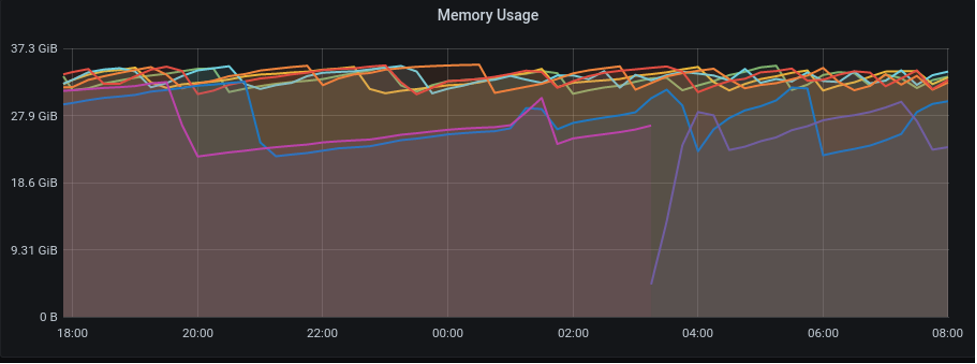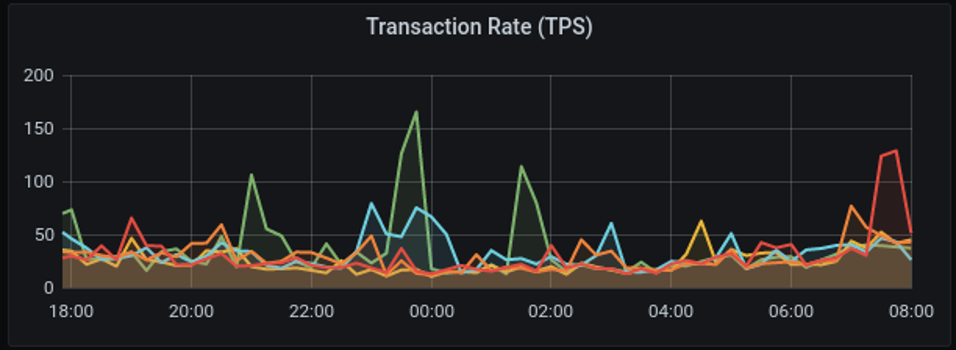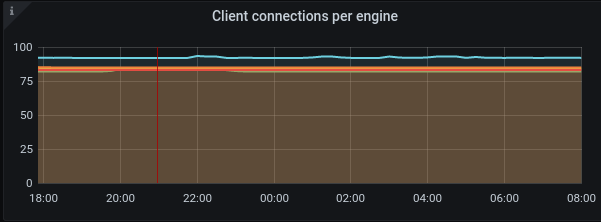# NuoDB Ops System Overview Graphs

This series of graphs are a collection of duplicate graphs for overview purposes.

## Memory usage

This graph is a duplicate of the Memory usage graph.Figure 1. Memory usage

### Use Case

Memory used will tend to the set memory value for the `--mem` option. There will be a saw-tooth pattern where garbage collection will run. Flat lines must be investigated.

### Graph Creation Detail

To create a graph using other tools, use the following detail:

• Memory Usage: for the raw of `HeapAllocated`, average

## CPU utilization per engine

This graph plots the total CPU used per Storage Manager (SM) and Transaction Engine (TE) normalized to max 100%.Figure 2. CPU utilization per engine

### Use Case

The CPU usage per engine should not exceed 80% of max CPU. Anything over 80% must be investigated.

### Graph Creation Detail

To create a graph using other tools, use the following detail:

• PercentCpuTime: for the norm of `PercentCpuTime`, average

## Transaction rate

This graph plots the rate of transactions committed per second. This is similar to Commits in SQL group of graphs.Figure 3. Transaction rate

### Use Case

Typically, the transaction rate depends on the workload. A heavier workload will mean a higher transaction rate. Longer periods where individual TEs have a high transaction rate may need investigation.

### Graph Creation Detail

To create a graph using other tools, use the following detail:

• Transaction rate: for the rate of Commits for TEs, average

## Aggregate transaction rate

The sum of all the transactions per second across all TEs and the total number of TEs.Figure 4. Aggregate Transaction rate

### Use Case

Typically, the aggregate transaction rate depends on the workload. A heavier workload will mean a higher aggregate transaction rate. Longer periods where there is a high aggregate transaction rate may need investigation.

### Graph Creation Details

To create a graph using other tools, use the following details:

• Aggregate Transaction Rate: sum the rate of Commits from each TE

• Number of TEs: sum the value of Milliseconds from each TE

## Client connections per engine

The number of client connections per TE. Similar to Connections in SQL graphs.Figure 5. Client Connections per Engine

### Use case

Typically, client connections per SM and TE depend on application configuration and should be evenly spread across all TEs (except for tie-breaker TEs).

### Graph Creation Detail

To create a graph using other tools, use the following detail:

• client connections: for the raw number of ClientCncts, average.